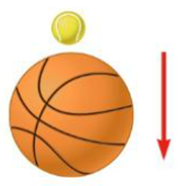Chapter 6, Problem 45P

Chapter
Section
Textbook Problem

A tennis ball of mass 57.0 g is held just above a basketball of mass 590 g. With their centers vertically aligned, both balls are released from rest at the same time, falling through a distance of 1.20 m, as shown in Figure P6.45. (a) Find the magnitude of the basketball’s velocity the instant before the basketball reaches the ground. (b) Assume that an elastic collision with the ground instantaneously reverses the velocity of the basketball so that it collides with the tennis ball just above it. To what height does the tennis ball rebound?Figure P6.45

(a)

To determine
The magnitude of the basketball’s velocity the instant before the basketball reaches the ground.

Explanation

The velocity of both balls just before the basketball reaches the ground is,

v=v0y2+2ayΔy=v0y2+2(g)(h)

• v0y is the initial velocity in vertical direction
• g is the acceleration due to gravity
• h is the height

Substitute 0m/s for v0y , 9

(b)

To determine
The height to which the tennis ball rebound.

Still sussing out bartleby?

Check out a sample textbook solution.

See a sample solution

The Solution to Your Study Problems

Bartleby provides explanations to thousands of textbook problems written by our experts, many with advanced degrees!

Get Started